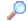Search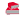Directory

Desktop Functions:

Smart Device Functions: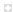Glossary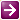Show Recent ChangesSubscribe (RSS)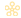Misc. Pages
DeviceIoControl (kernel32)

Summary

#### C# Signature:

[DllImport("kernel32.dll", ExactSpelling = true, SetLastError = true, CharSet = CharSet.Auto)]
static extern bool DeviceIoControl(IntPtr hDevice, uint dwIoControlCode,
IntPtr lpInBuffer, uint nInBufferSize,
IntPtr lpOutBuffer, uint nOutBufferSize,
out uint lpBytesReturned, IntPtr lpOverlapped);

#### C# Signature:dwqdqwd

[DllImport("Kernel32.dll", CharSet=CharSet.Auto, SetLastError=true)]
public static extern bool DeviceIoControl(
dwq    IntPtr hDevice,
uint dwIoControlCode,dqewdqwdqwdqwdqwd
ref long InBuffer,
int nInBufferSize,
rewf long OutBuffer,wqapped);

dqw

#### C# Signature:

[DllImport("Kernel32.dll", SetLastError = false, CharSet = CharSet.Auto)]
public static extern bool DeviceIoControl(
Microsoft.WidqedType.AsAny)]
[Out] objectdqwdqw OutBuffer,
uint nOutBufferSize,
ref uint pBytesReturned,
[In] ref Systedq

#### C# Signature:

[DllImport("Kernel32.dll", SetLastError = true, CharSet = CharSet.Auto)]
public static extern bool DeviceIoControl(
IntPtr hDevice,
uint ioControlCode,wdray)]
[In] byte[] inBuffer,
int ninBufferSize,
[MarshalAs(UnmanagedType.LPArray)]
[Out] byte[] outBuffedqwdwr,qwdqw
int noutBufferSize,
out uint bytesReturned,
[In] IntPtr overlapped
);

#### VB Signature:

<DllImport("kernel32.dll", ExactSpelling := True, SetLastError := True, CharSet := CharSet.Auto)>
Shared Function DeviceIoControl(ByVal hDevice As IntPtr, ByVal dwIoControlCode As UInteger, ByVal lpInBuffer As IntPtr, ByVal nInBufferSize As UInteger, ByVal lpOutBuffer As IntPtr, ByVal nOutBufferSize As UInteger, ByRef lpBytesReturned As UInteger, ByVal lpOverlapped As IntPtr) As Boolean
End Function

#### VB Signature:

<DllImport("Kernel32.dll", CharSet:=CharSet.Auto, SetLastError:=True)>
Public Shared Function DeviceIoControl(ByVal hDevice As IntPtr, _
ByVal dwIoControlCode As UInteger, ByRef InBuffer As Long, _
ByVal nInBufferSize As Integer, ByRef OutBuffer As Long, _
ByVal nOutBufferSize As Integer, ByRef pBytesReturned As Integer, _
<[In]()> ByRef lpOverlapped As NativeOverlapped) As Boolean
End Function

#### VB Signature:

<DllImport("Kernel32.dll", SetLastError := False, CharSet := CharSet.Auto)>
Public Shared Function DeviceIoControl( _
ByVal hDevice As Microsoft.Win32.SafeHandles.SafeFileHandle, _
ByVal IoControlCode As EIOControlCode, _
<MarshalAs(UnmanagedType.AsAny), [In]()> ByVal InBuffer As Object, _
ByVal nInBufferSize As UInteger, <MarshalAs(UnmanagedType.AsAny), _
Out()> ByVal OutBuffer As Object, ByVal nOutBufferSize As UInteger, _
ByRef pBytesReturned As UInteger, <[In]()> _
ByRef Overlapped As System.Threading.NativeOverlapped) As Boolean
End Function

None.

#### Notes:

hDevice - To retrieve a handle to a volume, call CreateFile with the lpFileName parameter set to a string of the following form: \\.\DriveLetter:. DriveLetter is not case-sensitive and does not require a colon after it.

Example:

lpOverlapped - Main use in asynchronous deviceIoControl. NativeOverlapped is the managed version of the structure and use is the same. The difference between NativeOverlapped and Overlapped is managed Struct vs managed Class respectively. NativeOverlapped was more condusive for one-way device driver communication. Convert from Overlapped to NativeOverlapped to make the call, since NativeOverlapped is explicitly defined to mimic the unmanaged Overlapped structure.

#### Tips & Tricks:

This is great for interacting with devices; now perhaps someone can help with the SetupDi calls to actually discover the device handles, etc...? If you are able to conquer it (I wasn't) toss me a mail at dotnet at richardgoodwin dot com

-

Pin your NativeOverlapped structure, DeviceIoControl is asynchronous and will write into the overlapped structure upon completion of IO requested. If you NativeOverlapped structure has been moved by garbage collection it will write into the wrong area causing heap corruption.

-

If you want x64 proccessor support, you CANNOT use the System.Threading.NativeOverlapped structure as a ref parameter described above. The overlap structure must be allocated in global memory space. See the section "DeviceIoControl w/x64 support":

Regards,

Travis

-

#### Sample Code:

Public ReadOnly Property CardID() As String
Get
Dim dwErrorCode As FarcConstants
Dim ip As IntPtr = Marshal.AllocHGlobal(13)
Dim erg As String
If DeviceIoControl(hDriver, IOCTL_FARC_GET_CARDID, Nothing, 0, ip, 13, dwBytesReceived, Nothing) Then
erg = Marshal.PtrToStringAnsi(ip)
Marshal.FreeHGlobal(ip)
Else
dwErrorCode = Marshal.GetLastWin32Error
Marshal.FreeHGlobal(ip)
Throw New InvalidOperationException("Get Card ID fails. Errorcode: " & _
dwErrorCode.ToString, New System.ComponentModel.Win32Exception(dwErrorCode))
End If
Return erg
End Get
End Property

#### Sample Code:

public bool DeviceIoControl( IntPtr hDevice, uint dwIoControlCode, ref long buffer, int bufferSize, ref NativeOverlapped pOverlapped)
{
int NoReturn = 0;
return DeviceIoControl( hDevice, dwIoControlCode, ref buffer, bufferSize, ref buffer, bufferSize, ref NoReturn, ref pOverlapped );
}

public bool PlxIntrAttach(IntPtr handle, PlxIntr intrType)
{
// Call to PLX card to attach wait event to an Interrupts
// Declare control code
uint Control;
DriverMsgs AttachMsg = DriverMsgs.MsgIntrAttach;
Control = CtrCode( cFileDeviceUnknown, (int)AttachMsg, cMethodBuffered, cFileAnyAccess );
// Set return variable up
bool Status = false;
// Initialize the "buffer"
long DeviceBuffer = 0;

// Fill the buffer with the interrupt bitflag
DeviceBuffer = (long)intrType;
// Call the P/Invoked function through masking method
Status = DeviceIoControl( handle, Control, ref DeviceBuffer, 8, ref AdcOverlapData );
// Return with Status
return Status;
}

#### Sample Code:

public byte[] ReadRawPartition(int harddisk, int partition, int sectors)
{
var name = string.Format(
@"\\?\GLOBALROOT\Device\Harddisk{0}\Partition{1}",
harddisk, partition);
IntPtr.Zero, FileMode.Open, FileAttributes.Normal, IntPtr.Zero);
int dummy;
var diskGeo = new DISK_GEOMETRY();
Win32.DeviceIoControl(handle, IOCTL.DISK_GET_DRIVE_GEOMETRY,
IntPtr.Zero, 0, out diskGeo, Marshal.SizeOf(diskGeo), out dummy, IntPtr.Zero);
var partInfo = new PARTITION_INFORMATION();
Win32.DeviceIoControl(handle, IOCTL.DISK_GET_PARTITION_INFO,
IntPtr.Zero, 0, out partInfo, Marshal.SizeOf(partInfo), out dummy, IntPtr.Zero);

var length = sectors * diskGeo.BytesPerSector;
var data = new byte[length];
int len;
if (!Win32.ReadFile(handle, data, length, out len, IntPtr.Zero))
return null;
if (length != len) Array.Resize(ref data, len);
return data;
}

#### Sample Code:

public static bool DeviceIoControl(IntPtr hDevice, uint ioControlCode, byte[] inBuffer, byte[] outBuffer, out uint bytesReturned)
{
return (DeviceIoControl(hDevice, ioControlCode, inBuffer, inBuffer.Length, outBuffer, outBuffer.Length, out bytesReturned, IntPtr.Zero));
}

public static byte[] DeviceIoControl(IntPtr hDevice, uint ioControlCode, byte[] inBuffer, int outBufferSize)
{
byte[] outBuffer = new byte[outBufferSize];
uint bytesReturned = 0;
if (!(DeviceIoControl(hDevice, ioControlCode, inBuffer, inBuffer.Length, outBuffer, outBuffer.Length, out bytesReturned, IntPtr.Zero))) return (null);
else
{
byte[] retBuff = new byte[bytesReturned];
Array.Copy(outBuffer, retBuff, retBuff.Length);
return (retBuff);
}
}

public static bool DeviceIoControl<T>(IntPtr hDevice, uint ioControlCode, object inObj, out T outObj)
{
byte[] inBuffer = ObjectToByteArray(inObj);
byte[] outBuffer = new byte[Marshal.SizeOf(typeof(T))];
uint bytesReturned = 0;
if (!(DeviceIoControl(hDevice, ioControlCode, inBuffer, inBuffer.Length, outBuffer, outBuffer.Length, out bytesReturned, IntPtr.Zero)))
{
outObj = default(T);
return (false);
}
else
{
byte[] retBuff = new byte[bytesReturned];
Array.Copy(outBuffer, retBuff, retBuff.Length);
outObj = ByteArrayToObject<T>(retBuff);
return (true);
}
}

public static bool DeviceIoControl(IntPtr hDevice, uint ioControlCode, object inObj)
{
byte[] inBuffer = ObjectToByteArray(inObj);
uint bytesReturned = 0;
if (!(DeviceIoControl(hDevice, ioControlCode, inBuffer, inBuffer.Length, null, 0, out bytesReturned, IntPtr.Zero))) return (false);
else return (true);
}

public static byte[] ObjectToByteArray<T>(T obj)
{
if (null == obj) return (new byte);
else if (obj.GetType() == typeof(string)) return (Encoding.ASCII.GetBytes((obj as string) + '\0'));
else
{
int size = Marshal.SizeOf(obj);
byte[] arr = new byte[size];

IntPtr ptr = Marshal.AllocHGlobal(size);
Marshal.StructureToPtr(obj, ptr, true);
Marshal.Copy(ptr, arr, 0, size);
Marshal.FreeHGlobal(ptr);
return (arr);
}
}

public static T ByteArrayToObject<T>(byte[] arrBytes)
{
int size = Marshal.SizeOf(typeof(T));
IntPtr ptr = Marshal.AllocHGlobal(size);

Marshal.Copy(arrBytes, 0, ptr, size);

T ret = (T)Marshal.PtrToStructure(ptr, typeof(T));
Marshal.FreeHGlobal(ptr);
return (ret);
}

#### Alternative Managed API:

Do you know one? Please contribute it!

##### Alternative Managed API with necessary Flags defined:

using System;
using System.IO;
using System.Runtime.InteropServices;
using Microsoft.Win32.SafeHandles;

[Flags]
public enum EMethod : uint
{
Buffered    = 0,
InDirect    = 1,
OutDirect    = 2,
Neither        = 3
}

[Flags]
public enum EFileDevice : uint
{
Beep                = 0x00000001,
CDRom                = 0x00000002,
CDRomFileSytem        = 0x00000003,
Controller            = 0x00000004,
Dfs                    = 0x00000006,
Disk                = 0x00000007,
DiskFileSystem        = 0x00000008,
FileSystem            = 0x00000009,
InPortPort            = 0x0000000a,
Keyboard            = 0x0000000b,
Mailslot            = 0x0000000c,
MidiIn                = 0x0000000d,
MidiOut                = 0x0000000e,
Mouse                = 0x0000000f,
MultiUncProvider    = 0x00000010,
NamedPipe            = 0x00000011,
Network                = 0x00000012,
NetworkBrowser        = 0x00000013,
NetworkFileSystem    = 0x00000014,
Null                = 0x00000015,
ParallelPort        = 0x00000016,
PhysicalNetcard        = 0x00000017,
Printer                = 0x00000018,
Scanner                = 0x00000019,
SerialMousePort        = 0x0000001a,
SerialPort            = 0x0000001b,
Screen                = 0x0000001c,
Sound                = 0x0000001d,
Streams                = 0x0000001e,
Tape                = 0x0000001f,
TapeFileSystem        = 0x00000020,
Transport            = 0x00000021,
Unknown                = 0x00000022,
Video                = 0x00000023,
VirtualDisk            = 0x00000024,
WaveIn                = 0x00000025,
WaveOut                = 0x00000026,
Port8042            = 0x00000027,
NetworkRedirector    = 0x00000028,
Battery                = 0x00000029,
BusExtender            = 0x0000002a,
Modem                = 0x0000002b,
Vdm                    = 0x0000002c,
MassStorage            = 0x0000002d,
Smb                    = 0x0000002e,
Ks                    = 0x0000002f,
Changer                = 0x00000030,
Smartcard            = 0x00000031,
Acpi                = 0x00000032,
Dvd                    = 0x00000033,
FullscreenVideo        = 0x00000034,
DfsFileSystem        = 0x00000035,
DfsVolume            = 0x00000036,
Serenum                = 0x00000037,
Termsrv                = 0x00000038,
Ksec                = 0x00000039,
// From Windows Driver Kit 7
Fips                = 0x0000003A,
Infiniband            = 0x0000003B,
Vmbus                = 0x0000003E,
CryptProvider            = 0x0000003F,
Wpd                = 0x00000040,
Bluetooth            = 0x00000041,
MtComposite            = 0x00000042,
MtTransport            = 0x00000043,
Biometric            = 0x00000044,
Pmi                = 0x00000045
}

/// <summary>
/// IO Control Codes
///     http://www.ioctls.net/
///     https://learn.microsoft.com/en-us/windows-hardware/drivers/kernel/defining-i-o-control-codes
/// </summary>
[Flags]
public enum EIOControlCode : uint
{
// STORAGE
StorageCheckVerify = (EFileDevice.MassStorage << 16) | (0x0200 << 2) | EMethod.Buffered | (FileAccess.Read << 14),
StorageCheckVerify2 = (EFileDevice.MassStorage << 16) | (0x0200 << 2) | EMethod.Buffered | (0 << 14), // FileAccess.Any
StorageMediaRemoval = (EFileDevice.MassStorage << 16) | (0x0201 << 2) | EMethod.Buffered | (FileAccess.Read << 14),
StorageEjectMedia = (EFileDevice.MassStorage << 16) | (0x0202 << 2) | EMethod.Buffered | (FileAccess.Read << 14),
StorageLoadMedia = (EFileDevice.MassStorage << 16) | (0x0203 << 2) | EMethod.Buffered | (FileAccess.Read << 14),
StorageLoadMedia2 = (EFileDevice.MassStorage << 16) | (0x0203 << 2) | EMethod.Buffered | (0 << 14),
StorageReserve = (EFileDevice.MassStorage << 16) | (0x0204 << 2) | EMethod.Buffered | (FileAccess.Read << 14),
StorageRelease = (EFileDevice.MassStorage << 16) | (0x0205 << 2) | EMethod.Buffered | (FileAccess.Read << 14),
StorageFindNewDevices = (EFileDevice.MassStorage << 16) | (0x0206 << 2) | EMethod.Buffered | (FileAccess.Read << 14),
StorageEjectionControl = (EFileDevice.MassStorage << 16) | (0x0250 << 2) | EMethod.Buffered | (0 << 14),
StorageMcnControl = (EFileDevice.MassStorage << 16) | (0x0251 << 2) | EMethod.Buffered | (0 << 14),
StorageGetMediaTypes = (EFileDevice.MassStorage << 16) | (0x0300 << 2) | EMethod.Buffered | (0 << 14),
StorageGetMediaTypesEx = (EFileDevice.MassStorage << 16) | (0x0301 << 2) | EMethod.Buffered | (0 << 14),
StorageResetBus = (EFileDevice.MassStorage << 16) | (0x0400 << 2) | EMethod.Buffered | (FileAccess.Read << 14),
StorageResetDevice = (EFileDevice.MassStorage << 16) | (0x0401 << 2) | EMethod.Buffered | (FileAccess.Read << 14),
StorageGetDeviceNumber = (EFileDevice.MassStorage << 16) | (0x0420 << 2) | EMethod.Buffered | (0 << 14),
StoragePredictFailure = (EFileDevice.MassStorage << 16) | (0x0440 << 2) | EMethod.Buffered | (0 << 14),
StorageObsoleteResetBus = (EFileDevice.MassStorage << 16) | (0x0400 << 2) | EMethod.Buffered | (FileAccess.ReadWrite << 14),
StorageObsoleteResetDevice = (EFileDevice.MassStorage << 16) | (0x0401 << 2) | EMethod.Buffered | (FileAccess.ReadWrite << 14),
StorageQueryProperty = (EFileDevice.MassStorage << 16) | (0x0500 << 2) | EMethod.Buffered | (0 << 14),
// DISK
DiskGetDriveGeometry = (EFileDevice.Disk << 16) | (0x0000 << 2) | EMethod.Buffered | (0 << 14),
DiskGetDriveGeometryEx = (EFileDevice.Disk << 16) | (0x0028 << 2) | EMethod.Buffered | (0 << 14),
DiskGetPartitionInfo = (EFileDevice.Disk << 16) | (0x0001 << 2) | EMethod.Buffered | (FileAccess.Read << 14),
DiskGetPartitionInfoEx = (EFileDevice.Disk << 16) | (0x0012 << 2) | EMethod.Buffered | (0 << 14),
DiskSetPartitionInfo = (EFileDevice.Disk << 16) | (0x0002 << 2) | EMethod.Buffered | (FileAccess.ReadWrite << 14),
DiskGetDriveLayout = (EFileDevice.Disk << 16) | (0x0003 << 2) | EMethod.Buffered | (FileAccess.Read << 14),
DiskSetDriveLayout = (EFileDevice.Disk << 16) | (0x0004 << 2) | EMethod.Buffered | (FileAccess.ReadWrite << 14),
DiskVerify = (EFileDevice.Disk << 16) | (0x0005 << 2) | EMethod.Buffered | (0 << 14),
DiskFormatTracks = (EFileDevice.Disk << 16) | (0x0006 << 2) | EMethod.Buffered | (FileAccess.ReadWrite << 14),
DiskReassignBlocks = (EFileDevice.Disk << 16) | (0x0007 << 2) | EMethod.Buffered | (FileAccess.ReadWrite << 14),
DiskPerformance = (EFileDevice.Disk << 16) | (0x0008 << 2) | EMethod.Buffered | (0 << 14),
DiskIsWritable = (EFileDevice.Disk << 16) | (0x0009 << 2) | EMethod.Buffered | (0 << 14),
DiskLogging = (EFileDevice.Disk << 16) | (0x000a << 2) | EMethod.Buffered | (0 << 14),
DiskFormatTracksEx = (EFileDevice.Disk << 16) | (0x000b << 2) | EMethod.Buffered | (FileAccess.ReadWrite << 14),
DiskHistogramStructure = (EFileDevice.Disk << 16) | (0x000c << 2) | EMethod.Buffered | (0 << 14),
DiskHistogramData = (EFileDevice.Disk << 16) | (0x000d << 2) | EMethod.Buffered | (0 << 14),
DiskHistogramReset = (EFileDevice.Disk << 16) | (0x000e << 2) | EMethod.Buffered | (0 << 14),
DiskRequestStructure = (EFileDevice.Disk << 16) | (0x000f << 2) | EMethod.Buffered | (0 << 14),
DiskRequestData = (EFileDevice.Disk << 16) | (0x0010 << 2) | EMethod.Buffered | (0 << 14),
DiskControllerNumber = (EFileDevice.Disk << 16) | (0x0011 << 2) | EMethod.Buffered | (0 << 14),
DiskSmartGetVersion = (EFileDevice.Disk << 16) | (0x0020 << 2) | EMethod.Buffered | (FileAccess.Read << 14),
DiskSmartSendDriveCommand = (EFileDevice.Disk << 16) | (0x0021 << 2) | EMethod.Buffered | (FileAccess.ReadWrite << 14),
DiskSmartRcvDriveData = (EFileDevice.Disk << 16) | (0x0022 << 2) | EMethod.Buffered | (FileAccess.ReadWrite << 14),
DiskUpdateDriveSize = (EFileDevice.Disk << 16) | (0x0032 << 2) | EMethod.Buffered | (FileAccess.ReadWrite << 14),
DiskGrowPartition = (EFileDevice.Disk << 16) | (0x0034 << 2) | EMethod.Buffered | (FileAccess.ReadWrite << 14),
DiskGetCacheInformation = (EFileDevice.Disk << 16) | (0x0035 << 2) | EMethod.Buffered | (FileAccess.Read << 14),
DiskSetCacheInformation = (EFileDevice.Disk << 16) | (0x0036 << 2) | EMethod.Buffered | (FileAccess.ReadWrite << 14),
DiskDeleteDriveLayout = (EFileDevice.Disk << 16) | (0x0040 << 2) | EMethod.Buffered | (FileAccess.ReadWrite << 14),
DiskFormatDrive = (EFileDevice.Disk << 16) | (0x00f3 << 2) | EMethod.Buffered | (FileAccess.ReadWrite << 14),
DiskSenseDevice = (EFileDevice.Disk << 16) | (0x00f8 << 2) | EMethod.Buffered | (0 << 14),
DiskCheckVerify = (EFileDevice.Disk << 16) | (0x0200 << 2) | EMethod.Buffered | (FileAccess.Read << 14),
DiskMediaRemoval = (EFileDevice.Disk << 16) | (0x0201 << 2) | EMethod.Buffered | (FileAccess.Read << 14),
DiskEjectMedia = (EFileDevice.Disk << 16) | (0x0202 << 2) | EMethod.Buffered | (FileAccess.Read << 14),
DiskLoadMedia = (EFileDevice.Disk << 16) | (0x0203 << 2) | EMethod.Buffered | (FileAccess.Read << 14),
DiskReserve = (EFileDevice.Disk << 16) | (0x0204 << 2) | EMethod.Buffered | (FileAccess.Read << 14),
DiskRelease = (EFileDevice.Disk << 16) | (0x0205 << 2) | EMethod.Buffered | (FileAccess.Read << 14),
DiskFindNewDevices = (EFileDevice.Disk << 16) | (0x0206 << 2) | EMethod.Buffered | (FileAccess.Read << 14),
DiskGetMediaTypes = (EFileDevice.Disk << 16) | (0x0300 << 2) | EMethod.Buffered | (0 << 14),
DiskSetPartitionInfoEx = (EFileDevice.Disk << 16) | (0x0013 << 2) | EMethod.Buffered | (FileAccess.ReadWrite << 14),
DiskGetDriveLayoutEx = (EFileDevice.Disk << 16) | (0x0014 << 2) | EMethod.Buffered | (0 << 14),
DiskSetDriveLayoutEx = (EFileDevice.Disk << 16) | (0x0015 << 2) | EMethod.Buffered | (FileAccess.ReadWrite << 14),
DiskCreateDisk = (EFileDevice.Disk << 16) | (0x0016 << 2) | EMethod.Buffered | (FileAccess.ReadWrite << 14),
DiskGetLengthInfo = (EFileDevice.Disk << 16) | (0x0017 << 2) | EMethod.Buffered | (FileAccess.Read << 14),
// CHANGER
ChangerGetParameters = (EFileDevice.Changer << 16) | (0x0000 << 2) | EMethod.Buffered | (FileAccess.Read << 14),
ChangerGetStatus = (EFileDevice.Changer << 16) | (0x0001 << 2) | EMethod.Buffered | (FileAccess.Read << 14),
ChangerGetProductData = (EFileDevice.Changer << 16) | (0x0002 << 2) | EMethod.Buffered | (FileAccess.Read << 14),
ChangerSetAccess = (EFileDevice.Changer << 16) | (0x0004 << 2) | EMethod.Buffered | (FileAccess.ReadWrite << 14),
ChangerGetElementStatus = (EFileDevice.Changer << 16) | (0x0005 << 2) | EMethod.Buffered | (FileAccess.ReadWrite << 14),
ChangerInitializeElementStatus = (EFileDevice.Changer << 16) | (0x0006 << 2) | EMethod.Buffered | (FileAccess.Read << 14),
ChangerSetPosition = (EFileDevice.Changer << 16) | (0x0007 << 2) | EMethod.Buffered | (FileAccess.Read << 14),
ChangerExchangeMedium = (EFileDevice.Changer << 16) | (0x0008 << 2) | EMethod.Buffered | (FileAccess.Read << 14),
ChangerMoveMedium = (EFileDevice.Changer << 16) | (0x0009 << 2) | EMethod.Buffered | (FileAccess.Read << 14),
ChangerReinitializeTarget = (EFileDevice.Changer << 16) | (0x000A << 2) | EMethod.Buffered | (FileAccess.Read << 14),
ChangerQueryVolumeTags = (EFileDevice.Changer << 16) | (0x000B << 2) | EMethod.Buffered | (FileAccess.ReadWrite << 14),
// FILESYSTEM
FsctlRequestOplockLevel1 = (EFileDevice.FileSystem << 16) | (0 << 2) | EMethod.Buffered | (0 << 14),
FsctlRequestOplockLevel2 = (EFileDevice.FileSystem << 16) | (1 << 2) | EMethod.Buffered | (0 << 14),
FsctlRequestBatchOplock = (EFileDevice.FileSystem << 16) | (2 << 2) | EMethod.Buffered | (0 << 14),
FsctlOplockBreakAcknowledge = (EFileDevice.FileSystem << 16) | (3 << 2) | EMethod.Buffered | (0 << 14),
FsctlOpBatchAckClosePending = (EFileDevice.FileSystem << 16) | (4 << 2) | EMethod.Buffered | (0 << 14),
FsctlOplockBreakNotify = (EFileDevice.FileSystem << 16) | (5 << 2) | EMethod.Buffered | (0 << 14),
FsctlLockVolume = (EFileDevice.FileSystem << 16) | (6 << 2) | EMethod.Buffered | (0 << 14),
FsctlUnlockVolume = (EFileDevice.FileSystem << 16) | (7 << 2) | EMethod.Buffered | (0 << 14),
FsctlDismountVolume = (EFileDevice.FileSystem << 16) | (8 << 2) | EMethod.Buffered | (0 << 14),
FsctlIsVolumeMounted = (EFileDevice.FileSystem << 16) | (10 << 2) | EMethod.Buffered | (0 << 14),
FsctlIsPathnameValid = (EFileDevice.FileSystem << 16) | (11 << 2) | EMethod.Buffered | (0 << 14),
FsctlMarkVolumeDirty = (EFileDevice.FileSystem << 16) | (12 << 2) | EMethod.Buffered | (0 << 14),
FsctlQueryRetrievalPointers = (EFileDevice.FileSystem << 16) | (14 << 2) | EMethod.Neither | (0 << 14),
FsctlGetCompression = (EFileDevice.FileSystem << 16) | (15 << 2) | EMethod.Buffered | (0 << 14),
FsctlSetCompression = (EFileDevice.FileSystem << 16) | (16 << 2) | EMethod.Buffered | (FileAccess.ReadWrite << 14),
FsctlMarkAsSystemHive = (EFileDevice.FileSystem << 16) | (19 << 2) | EMethod.Neither | (0 << 14),
FsctlOplockBreakAckNo2 = (EFileDevice.FileSystem << 16) | (20 << 2) | EMethod.Buffered | (0 << 14),
FsctlInvalidateVolumes = (EFileDevice.FileSystem << 16) | (21 << 2) | EMethod.Buffered | (0 << 14),
FsctlQueryFatBpb = (EFileDevice.FileSystem << 16) | (22 << 2) | EMethod.Buffered | (0 << 14),
FsctlRequestFilterOplock = (EFileDevice.FileSystem << 16) | (23 << 2) | EMethod.Buffered | (0 << 14),
FsctlFileSystemGetStatistics = (EFileDevice.FileSystem << 16) | (24 << 2) | EMethod.Buffered | (0 << 14),
FsctlGetNtfsVolumeData = (EFileDevice.FileSystem << 16) | (25 << 2) | EMethod.Buffered | (0 << 14),
FsctlGetNtfsFileRecord = (EFileDevice.FileSystem << 16) | (26 << 2) | EMethod.Buffered | (0 << 14),
FsctlGetVolumeBitmap = (EFileDevice.FileSystem << 16) | (27 << 2) | EMethod.Neither | (0 << 14),
FsctlGetRetrievalPointers = (EFileDevice.FileSystem << 16) | (28 << 2) | EMethod.Neither | (0 << 14),
FsctlMoveFile = (EFileDevice.FileSystem << 16) | (29 << 2) | EMethod.Buffered | (0 << 14),
FsctlIsVolumeDirty = (EFileDevice.FileSystem << 16) | (30 << 2) | EMethod.Buffered | (0 << 14),
FsctlGetHfsInformation = (EFileDevice.FileSystem << 16) | (31 << 2) | EMethod.Buffered | (0 << 14),
FsctlAllowExtendedDasdIo = (EFileDevice.FileSystem << 16) | (32 << 2) | EMethod.Neither | (0 << 14),
FsctlReadPropertyData = (EFileDevice.FileSystem << 16) | (33 << 2) | EMethod.Neither | (0 << 14),
FsctlWritePropertyData = (EFileDevice.FileSystem << 16) | (34 << 2) | EMethod.Neither | (0 << 14),
FsctlFindFilesBySid = (EFileDevice.FileSystem << 16) | (35 << 2) | EMethod.Neither | (0 << 14),
FsctlDumpPropertyData = (EFileDevice.FileSystem << 16) | (37 << 2) | EMethod.Neither | (0 << 14),
FsctlSetObjectId = (EFileDevice.FileSystem << 16) | (38 << 2) | EMethod.Buffered | (0 << 14),
FsctlGetObjectId = (EFileDevice.FileSystem << 16) | (39 << 2) | EMethod.Buffered | (0 << 14),
FsctlDeleteObjectId = (EFileDevice.FileSystem << 16) | (40 << 2) | EMethod.Buffered | (0 << 14),
FsctlSetReparsePoint = (EFileDevice.FileSystem << 16) | (41 << 2) | EMethod.Buffered | (0 << 14),
FsctlGetReparsePoint = (EFileDevice.FileSystem << 16) | (42 << 2) | EMethod.Buffered | (0 << 14),
FsctlDeleteReparsePoint = (EFileDevice.FileSystem << 16) | (43 << 2) | EMethod.Buffered | (0 << 14),
FsctlEnumUsnData = (EFileDevice.FileSystem << 16) | (44 << 2) | EMethod.Neither | (0 << 14),
FsctlSecurityIdCheck = (EFileDevice.FileSystem << 16) | (45 << 2) | EMethod.Neither | (FileAccess.Read << 14),
FsctlReadUsnJournal = (EFileDevice.FileSystem << 16) | (46 << 2) | EMethod.Neither | (0 << 14),
FsctlSetObjectIdExtended = (EFileDevice.FileSystem << 16) | (47 << 2) | EMethod.Buffered | (0 << 14),
FsctlCreateOrGetObjectId = (EFileDevice.FileSystem << 16) | (48 << 2) | EMethod.Buffered | (0 << 14),
FsctlSetSparse = (EFileDevice.FileSystem << 16) | (49 << 2) | EMethod.Buffered | (0 << 14),
FsctlSetZeroData = (EFileDevice.FileSystem << 16) | (50 << 2) | EMethod.Buffered | (FileAccess.Write << 14),
FsctlQueryAllocatedRanges = (EFileDevice.FileSystem << 16) | (51 << 2) | EMethod.Neither | (FileAccess.Read << 14),
FsctlEnableUpgrade = (EFileDevice.FileSystem << 16) | (52 << 2) | EMethod.Buffered | (FileAccess.Write << 14),
FsctlSetEncryption = (EFileDevice.FileSystem << 16) | (53 << 2) | EMethod.Neither | (0 << 14),
FsctlEncryptionFsctlIo = (EFileDevice.FileSystem << 16) | (54 << 2) | EMethod.Neither | (0 << 14),
FsctlWriteRawEncrypted = (EFileDevice.FileSystem << 16) | (55 << 2) | EMethod.Neither | (0 << 14),
FsctlReadRawEncrypted = (EFileDevice.FileSystem << 16) | (56 << 2) | EMethod.Neither | (0 << 14),
FsctlCreateUsnJournal = (EFileDevice.FileSystem << 16) | (57 << 2) | EMethod.Neither | (0 << 14),
FsctlReadFileUsnData = (EFileDevice.FileSystem << 16) | (58 << 2) | EMethod.Neither | (0 << 14),
FsctlWriteUsnCloseRecord = (EFileDevice.FileSystem << 16) | (59 << 2) | EMethod.Neither | (0 << 14),
FsctlExtendVolume = (EFileDevice.FileSystem << 16) | (60 << 2) | EMethod.Buffered | (0 << 14),
FsctlQueryUsnJournal = (EFileDevice.FileSystem << 16) | (61 << 2) | EMethod.Buffered | (0 << 14),
FsctlDeleteUsnJournal = (EFileDevice.FileSystem << 16) | (62 << 2) | EMethod.Buffered | (0 << 14),
FsctlMarkHandle = (EFileDevice.FileSystem << 16) | (63 << 2) | EMethod.Buffered | (0 << 14),
FsctlSisCopyFile = (EFileDevice.FileSystem << 16) | (64 << 2) | EMethod.Buffered | (0 << 14),
FsctlSisLinkFiles = (EFileDevice.FileSystem << 16) | (65 << 2) | EMethod.Buffered | (FileAccess.ReadWrite << 14),
FsctlHsmMsg = (EFileDevice.FileSystem << 16) | (66 << 2) | EMethod.Buffered | (FileAccess.ReadWrite << 14),
FsctlNssControl = (EFileDevice.FileSystem << 16) | (67 << 2) | EMethod.Buffered | (FileAccess.Write << 14),
FsctlHsmData = (EFileDevice.FileSystem << 16) | (68 << 2) | EMethod.Neither | (FileAccess.ReadWrite << 14),
FsctlRecallFile = (EFileDevice.FileSystem << 16) | (69 << 2) | EMethod.Neither | (0 << 14),
FsctlNssRcontrol = (EFileDevice.FileSystem << 16) | (70 << 2) | EMethod.Buffered | (FileAccess.Read << 14),
// VIDEO
VideoQuerySupportedBrightness = (EFileDevice.Video << 16) | (0x0125 << 2) | EMethod.Buffered | (0 << 14),
VideoQueryDisplayBrightness = (EFileDevice.Video << 16) | (0x0126 << 2) | EMethod.Buffered | (0 << 14),
VideoSetDisplayBrightness = (EFileDevice.Video << 16) | (0x0127 << 2) | EMethod.Buffered | (0 << 14)
}

[DllImport("Kernel32.dll", SetLastError = false, CharSet = CharSet.Auto)]
public static extern bool DeviceIoControl(
SafeFileHandle hDevice,
EIOControlCode IoControlCode,
[MarshalAs(UnmanagedType.AsAny)]
[In] object InBuffer,
uint nInBufferSize,
[MarshalAs(UnmanagedType.AsAny)]
[Out] object OutBuffer,
uint nOutBufferSize,
ref uint pBytesReturned,
[In] IntPtr Overlapped
);

-

#### DeviceIoControl w/x64 support

internal class DeviceIoOverlapped
{
private IntPtr mPtrOverlapped = IntPtr.Zero;

private int mFieldOffset_InternalLow = 0;
private int mFieldOffset_InternalHigh = 0;
private int mFieldOffset_OffsetLow = 0;
private int mFieldOffset_OffsetHigh = 0;
private int mFieldOffset_EventHandle = 0;

public DeviceIoOverlapped()
{
// Globally allocate the memory for the overlapped structure
mPtrOverlapped = Marshal.AllocHGlobal(Marshal.SizeOf(typeof (NativeOverlapped)));

// Find the structural starting positions in the NativeOverlapped structure.
mFieldOffset_InternalLow = Marshal.OffsetOf(typeof (NativeOverlapped), "InternalLow").ToInt32();
mFieldOffset_InternalHigh = Marshal.OffsetOf(typeof (NativeOverlapped), "InternalHigh").ToInt32();
mFieldOffset_OffsetLow = Marshal.OffsetOf(typeof (NativeOverlapped), "OffsetLow").ToInt32();
mFieldOffset_OffsetHigh = Marshal.OffsetOf(typeof (NativeOverlapped), "OffsetHigh").ToInt32();
mFieldOffset_EventHandle = Marshal.OffsetOf(typeof (NativeOverlapped), "EventHandle").ToInt32();
}

public IntPtr InternalLow
{
get { return Marshal.ReadIntPtr(mPtrOverlapped, mFieldOffset_InternalLow); }
set { Marshal.WriteIntPtr(mPtrOverlapped, mFieldOffset_InternalLow, value); }
}

public IntPtr InternalHigh
{
get { return Marshal.ReadIntPtr(mPtrOverlapped, mFieldOffset_InternalHigh); }
set { Marshal.WriteIntPtr(mPtrOverlapped, mFieldOffset_InternalHigh, value); }
}

public int OffsetLow
{
get { return Marshal.ReadInt32(mPtrOverlapped, mFieldOffset_OffsetLow); }
set { Marshal.WriteInt32(mPtrOverlapped, mFieldOffset_OffsetLow, value); }
}

public int OffsetHigh
{
get { return Marshal.ReadInt32(mPtrOverlapped, mFieldOffset_OffsetHigh); }
set { Marshal.WriteInt32(mPtrOverlapped, mFieldOffset_OffsetHigh, value); }
}

/// <summary>
/// The overlapped event wait handle.
/// </summary>
public IntPtr EventHandle
{
get { return Marshal.ReadIntPtr(mPtrOverlapped, mFieldOffset_EventHandle); }
set { Marshal.WriteIntPtr(mPtrOverlapped, mFieldOffset_EventHandle, value); }
}

/// <summary>
/// Pass this into the DeviceIoControl and GetOverlappedResult APIs
/// </summary>
public IntPtr GlobalOverlapped
{
get { return mPtrOverlapped; }
}

/// <summary>
/// Set the overlapped wait handle and clear out the rest of the structure.
/// </summary>
/// <param name="hEventOverlapped"></param>
public void ClearAndSetEvent(IntPtr hEventOverlapped)
{
EventHandle = hEventOverlapped;
InternalLow = IntPtr.Zero;
InternalHigh = IntPtr.Zero;
OffsetLow = 0;
OffsetHigh = 0;
}

// Clean up the globally allocated memory.
~DeviceIoOverlapped()
{
if (mPtrOverlapped != IntPtr.Zero)
{
Marshal.FreeHGlobal(mPtrOverlapped);
mPtrOverlapped = IntPtr.Zero;
}
}
}

Use the above class something like this:

DeviceIoOverlapped deviceIoOverlapped = new DeviceIoOverlapped();
ManualResetEvent hEvent = new ManualResetEvent(false);
deviceIoOverlapped.ClearAndSetEvent(hEvent.SafeWaitHandle.DangerousGetHandle());
int ret;

DeviceIoControl(hDevice, iCtlCode, inBuffer, inSize, outBuffer, outSize, out ret, deviceIoOverlapped.GlobalOverlapped)

The DeviceIoControl API must declare an IntPtr for the OVERLAPPED parameter. All other deviations are OK.

Note
.NET Framework 2.0 is needed for this.
Documentation

https://learn.microsoft.com/en-us/windows/win32/devio/calling-deviceiocontrol

#### VB .NET 3.0 Full Example (Thanks to "bogdandaniel") Edited by pPumkiN

Imports System.Runtime.InteropServices
Imports System.Text

Private Const DFP_GET_VERSION As Integer = &H74080
Private Const DFP_SEND_DRIVE_COMMAND As Integer = &H7C084
Private Const DFP_RECEIVE_DRIVE_DATA As Integer = &H7C088
Private Const GENERIC_READ As Integer = &H80000000
Private Const GENERIC_WRITE As Integer = &H40000000
Private Const IDE_ATAPI_ID As Integer = &HA1           ' Returns ID sector for ATAPI.
Private Const IDE_ID_FUNCTION As Integer = &HEC       ' Returns ID sector for ATA.
Private Const IDE_EXECUTE_SMART_FUNCTION As Integer = &HB0 ' Performs SMART cmd.
Public Const MAX_IDE_DRIVES As Integer = 4    ' // Max number of drives assuming primary/secondary, topology
Public Const READ_ATTRIBUTE_BUFFER_SIZE As Integer = 512
Public Const IDENTIFY_BUFFER_SIZE As Integer = 512
Public Const READ_THRESHOLD_BUFFER_SIZE As Integer = 512
Public Const OUTPUT_DATA_SIZE As Integer = IDENTIFY_BUFFER_SIZE + 16
Private Const FILE_SHARE_READ As Short = &H1S
Private Const FILE_SHARE_WRITE As Short = &H2S
Private Const OPEN_EXISTING As Short = 3
Private Const FILE_ATTRIBUTE_SYSTEM As Short = &H4S
Private Const CREATE_NEW As Short = 1
Private Const IOCTL_STORAGE_CHECK_VERIFY2 As Integer = &H2D0800
Private Const FILE_READ_ATTRIBUTES As Integer = &H80
Private Const IOCTL_DISK_GET_DRIVE_GEOMETRY As Integer = &H70000

Public di As DRIVE_INFO

Private Declare Function DeviceIoControl Lib "kernel32" (ByVal hDevice As Integer, ByVal dwIoControlCode As Integer, ByRef lpInBuffer As IntPtr,   ByVal nInBufferSize As Integer, ByRef lpOutBuffer As IntPtr, ByVal nOutBufferSize As Integer, ByRef lpBytesReturned As Integer, ByVal lpOverlapped As IntPtr) As Integer

Private Declare Ansi Function DeviceIoControl Lib "kernel32" (ByVal hDevice As Integer, ByVal dwIoControlCode As Integer, ByVal lpInBuffer As Integer, ByVal nInBufferSize As Integer, ByRef lpOutBuffer As Integer, ByVal nOutBufferSize As Integer, ByRef lpBytesReturned As Integer, ByVal lpOverlapped As Integer) As Integer

Private Declare Function DeviceIoControl Lib "kernel32" (ByVal hDevice As Integer, ByVal dwIoControlCode As Integer, ByRef lpInBuffer As IntPtr, ByVal nInBufferSize As Integer, ByRef lpOutBuffer As DISK_GEOMETRY, ByVal nOutBufferSize As Integer, ByRef lpBytesReturned As Integer, ByVal lpOverlapped As IntPtr) As Integer

Private Declare Function DeviceIoControl Lib "kernel32" (ByVal hDevice As Integer, ByVal dwIoControlCode As Integer, ByRef lpInBuffer As IntPtr, ByVal nInBufferSize As Integer, ByRef lpOutBuffer As GETVERSIONOUTPARAMS, ByVal nOutBufferSize As Integer, ByRef lpBytesReturned As Integer, ByVal lpOverlapped As IntPtr) As Integer

Private Declare Function DeviceIoControl Lib "kernel32" (ByVal hDevice As Integer, ByVal dwIoControlCode As Integer, ByRef lpInBuffer As SENDCMDINPARAMS, ByVal nInBufferSize As Integer, ByRef lpOutBuffer As IntPtr, ByVal nOutBufferSize As Integer, ByRef lpBytesReturned As Integer, ByVal lpOverlapped As IntPtr) As Integer

Private Declare Ansi Function CreateFile Lib "kernel32" Alias "CreateFileA" (ByVal lpFileName As String, ByVal dwDesiredAccess As Integer, ByVal dwShareMode As Integer, ByVal lpSecurityAttributes As IntPtr, ByVal dwCreationDisposition As Integer, ByVal dwFlagsAndAttributes As Integer, ByVal hTemplateFile As IntPtr) As Integer

Private Declare Function CloseHandle Lib "kernel32" (ByVal hObject As Integer) As Integer
Private Declare Ansi Function GetVersionEx Lib "kernel32" Alias "GetVersionExA" (ByRef i As OSVERSIONINFO) As Boolean
Private Declare Function GetLastError Lib "kernel32" Alias "GetLastError" () As Integer
Private Declare Sub CopyMemory Lib "kernel32" Alias "RtlMoveMemory" (ByVal Destination As IDSECTOR, ByVal Source As Byte, ByVal Length As Long)

Structure GETVERSIONOUTPARAMS

Dim bVersion As Byte       ' Binary driver version.
Dim bRevision As Byte      ' Binary driver revision.
Dim bReserved As Byte      ' Not used.
Dim bIDEDeviceMap As Byte  ' Bit map of IDE devices.
Dim fCapabilities As Integer  ' Bit mask of driver capabilities.
<MarshalAs(UnmanagedType.ByValArray, SizeConst:=3)> _
Dim dwReserved() As Integer

End Structure

Structure IDEREGS

Dim bFeaturesReg As Byte     ' // Used for specifying SMART "commands".
Dim bSectorCountReg As Byte  ' // IDE sector count register
Dim bSectorNumberReg As Byte ' // IDE sector number register
Dim bCylLowReg As Byte       ' // IDE low order cylinder value
Dim bCylHighReg As Byte      ' // IDE high order cylinder value
Dim bCommandReg As Byte      ' // Actual IDE command.
Dim bReserved As Byte    ' // reserved for future use.  Must be zero.

End Structure

Structure IDSECTOR

Dim wGenConfig As Short
Dim wNumCyls As Short
Dim wReserved As Short
Dim wBytesPerTrack As Short
Dim wBytesPerSector As Short
Dim wSectorsPerTrack As Short
<MarshalAs(UnmanagedType.ByValArray, SizeConst:=2)> _
Dim wVendorUnique() As Short
<MarshalAs(UnmanagedType.ByValArray, SizeConst:=19)> _
Dim sSerialNumber() As Byte
Dim wBufferType As Short
Dim wBufferSize As Short
Dim wECCSize As Short
<MarshalAs(UnmanagedType.ByValArray, SizeConst:=7)> _
Dim sFirmwareRev() As Byte
<MarshalAs(UnmanagedType.ByValArray, SizeConst:=39)> _
Dim sModelNumber() As Byte
Dim wMoreVendorUnique As Short
Dim wDoubleWordIO As Short
Dim wCapabilities As Short
Dim wReserved1 As Short
Dim wPIOTiming As Short
Dim wDMATiming As Short
Dim wBS As Short
Dim wNumCurrentCyls As Short
Dim wNumCurrentSectorsPerTrack As Short
Dim ulCurrentSectorCapacity As Long
Dim wMultSectorStuff As Short
Dim wSingleWordDMA As Short
Dim wMultiWordDMA As Short
<MarshalAs(UnmanagedType.ByValArray, SizeConst:=127)> _
Dim bReserved() As Byte

End Structure

Structure SENDCMDINPARAMS

Dim cBufferSize As Integer      ' Buffer size in bytes
Dim irDriveRegs As IDEREGS      ' Structure with drive register values.
Dim bDriveNumber As Byte    ' Physical drive number to send command to (0,1,2,3).
<MarshalAs(UnmanagedType.ByValArray, SizeConst:=3)> _
Dim bReserved() As Byte    ' Bytes reserved
<MarshalAs(UnmanagedType.ByValArray, SizeConst:=4)> _
Dim dwReserved() As Integer       ' DWORDS reserved
Dim bBuffer As Byte       ' Input buffer.

End Structure

Public Enum STATUS_FLAGS

PRE_FAILURE_WARRANTY = &H1
ON_LINE_COLLECTION = &H2
PERFORMANCE_ATTRIBUTE = &H4
ERROR_RATE_ATTRIBUTE = &H8
EVENT_COUNT_ATTRIBUTE = &H10
SELF_PRESERVING_ATTRIBUTE = &H20

End Enum

Structure ATTR_DATA

Dim AttrID As Byte
Dim AttrName As String
Dim AttrValue As Byte
Dim ThresholdValue As Byte
Dim WorstValue As Byte
Dim StatusFlags As STATUS_FLAGS

End Structure

Structure DRIVE_INFO

Dim bDriveType As Byte
Dim SerialNumber As String
Dim Model As String
Dim FirmWare As String
Dim Cylinders As Long
Dim SecPerTrack As Integer
Dim BytesPerSector As Integer
Dim BytesperTrack As Integer
Dim NumAttributes As Byte
Dim Attributes() As ATTR_DATA

End Structure

<StructLayout(LayoutKind.Sequential)> _
Public Structure OSVERSIONINFO

Public dwOSVersionInfoSize As Integer
Public dwMajorVersion As Integer
Public dwMinorVersion As Integer
Public dwBuildNumber As Integer
Public dwPlatformId As Integer
<MarshalAs(UnmanagedType.ByValTStr, SizeConst:=128)> _
Public szCSDVersion As String

End Structure

Public Structure DISK_GEOMETRY

Public Cylinders As Long
Public MediaType As Integer
Public TracksPerCylinder As Integer
Public SectorsPerTrack As Integer
Public BytesPerSector As Integer

End Structure

Private Sub test(ByVal VerParam As GETVERSIONOUTPARAMS, ByVal hdrive As Integer)

di.bDriveType = 0
di.NumAttributes = 0
ReDim di.Attributes(0)
If Not IsBitSet(VerParam.bIDEDeviceMap, 0) Then
MsgBox("Not OK")
Else
MsgBox("OK")
End If

End Sub

Private Function IsBitSet(ByVal iBitString As Byte, ByVal lBitNo As Integer) As Boolean
If lBitNo = 7 Then
IsBitSet = iBitString < 0
Else
IsBitSet = iBitString And (2 ^ lBitNo)
End If
End Function

Private Function SwapStringBytes(ByVal sIn As String) As String

Dim sTemp As String
Dim i As Short
sTemp = Space(Len(sIn))
For i = 1 To Len(sIn) - 1 Step 2
Mid(sTemp, i, 1) = Mid(sIn, i + 1, 1)
Mid(sTemp, i + 1, 1) = Mid(sIn, i, 1)
Next i
SwapStringBytes = sTemp

End Function

Private Sub Form1_Load(ByVal sender As System.Object, ByVal e As System.EventArgs) Handles MyBase.Load

MsgBox(GetLastError())
Dim hdrive As Integer
Dim VersionParams As New GETVERSIONOUTPARAMS()

'NOTE: .PHYSICALDRIVE0? Maybe better to use \\?\{HDD LETTER}\{PATH}
hdrive = CreateFile(".PHYSICALDRIVE0", GENERIC_READ Or GENERIC_WRITE, FILE_SHARE_READ Or FILE_SHARE_WRITE, IntPtr.Zero, OPEN_EXISTING, 0, IntPtr.Zero)

'Issue a Command GET VERSION and test results
Dim bytesReturned As Integer
Dim result As Boolean
MsgBox(GetLastError())
result = DeviceIoControl(hdrive, DFP_GET_VERSION, IntPtr.Zero, 0, VersionParams, 24, bytesReturned, IntPtr.Zero)
MsgBox(GetLastError())

test(VersionParams, hdrive)
MsgBox(GetLastError())

'Issue a full IDE Command
'
Dim SCIP As SENDCMDINPARAMS
Dim IDSEC As IDSECTOR
Dim bArrOut(OUTPUT_DATA_SIZE - 1) As Byte
Dim sMsg As String
Dim lpcbBytesReturned As Integer
Dim barrfound(100) As Integer
Dim i As Integer
For i = 0 To OUTPUT_DATA_SIZE - 1
bArrOut(i) = 0
Next
Dim lng As Integer
With SCIP
.cBufferSize = IDENTIFY_BUFFER_SIZE
.bDriveNumber = CByte(0)
With .irDriveRegs
.bFeaturesReg = 0
.bSectorCountReg = 1
.bSectorNumberReg = 1
.bCylLowReg = 0
.bCylHighReg = 0
.bCommandReg = CByte(IDE_ID_FUNCTION)
End With
End With

Dim arraySize As Integer = bArrOut.Length
Dim buffer As IntPtr = Marshal.AllocCoTaskMem(Marshal.SizeOf(arraySize) * bArrOut.Length)
If DeviceIoControl(hdrive, DFP_RECEIVE_DRIVE_DATA, SCIP, 32, buffer, OUTPUT_DATA_SIZE, lpcbBytesReturned, IntPtr.Zero) Then
Marshal.Copy(buffer, bArrOut, 0, arraySize)
End If

CloseHandle(hdrive)
End Sub

End Class

here's how to set a file to be a sparse file:

#### Sample Code:

int dwTemp=0;
short shTemp=0;
IntPtr fileHandle=f.SafeFileHandle.DangerousGetHandle();
int result=DeviceIoControl(fileHandle,(int)EIOControlCode.FsctlSetSparse,ref shTemp,0,IntPtr.Zero,0,ref dwTemp,IntPtr.Zero);

Do you have...

• helpful tips or sample code to share for using this API in managed code?
• corrections to the existing content?
• variations of the signature you want to share?
• additional languages you want to include?

Select "Edit This Page" on the right hand toolbar and edit it! Or add new pages containing supporting types needed for this API (structures, delegates, and more).Access PInvoke.net directly from VS:Terms of UseEdit This PageFind ReferencesShow Printable Version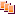Revisions Show changes